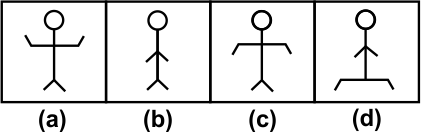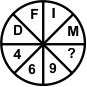# CREST Reasoning Olympiad Class 10 Sample Papers

REGISTER NOW

## Syllabus:

Section 1: Series Completion, Analogy and Classification, Coding - Decoding, Blood Relations, Sequential Output Tracing , Direction Sense Test, Logical Venn Diagrams, Alphabet Test and Logical, Alpha-Numeric, Number, Mathematical Operations, Inserting the Missing Characters, Analytical Reasoning, Mirror Images and Water Images, Spotting Out The Embedded, Figure Matrix, Paper Folding and Paper Cutting, Grouping of Identical Figures, Cubes and Dice, Dot Situation, Construction of Squares and Triangles, Figure Formation and Analysis.

Achievers Section: Higher Order Thinking Questions - Syllabus as per Section 1

 Q.1 Six friends are seated on a table built in the shape of a regular hexagon. B, C, E and F are seated relatively closer to A than D is from A. C and E are at the same distance from A. B is seated to the immediate left of C but not adjacent to D. Who is sitting between E and C?
 Q.2 In the following question, four figures are given. Three are similar in a certain way and so form a group. Find out which one of the figures does not belong that group.Q.3 Determine the term that would come in place of the question mark.Q.4 Which group of numbers has the same property of the numbers as in the given set? Given set: {48, 40, 24}
 Q.5 Five friends A, B, C, D and E go to watch a movie in a cinema hall. They sit in the front row on seats numbered 1-5, where 5 is to the extreme right. E sits to the immediate right of A, who doesn't sit on seat numbered 1. The sum of the seat numbers of C and D is equal to the sum of seat numbers of B and E. Which one of the five persons is sitting in the middle?
 Q.6 Study the following information carefully and answer the question given below:(i) 'pa ki top ma' means 'Usha is playing cards'.(ii) 'kop ja ki ma' means 'Asha is playing tennis'.(iii) 'ki top sop ha' means 'they are playing football'.(iv) 'pa sur kop' means 'card and tennis'. Which of the following represents 'tennis' in that language?
 Q.7 What comes in place of question mark (?) in the following letter series? PMK, MPK, MKP, KMP, ?
 Q.8 Vikas starts from point A and covers a distance of 2 km in South direction. He then turns to his left and covers 1 km, then he again turns to his left and covers 3 km to reach point B. In which direction he is from the starting point?
 Q.9 If 'A' is coded as 2, 'B' as 4, 'C' as 6 and so on, then which of the following is the numerical value of the word 'ORANGE'?
 Q.10 In the given question, a series of letters and numbers is given, the terms of which follow a certain pattern in groups. However, some terms in the series are missing, which are given in the same order as one of the alternatives below the series. Choose the correct alternatives. TP_16_N18_PL_12N_1410

Sample PDF of CREST Reasoning Olympiad for Class 10: